# Coaching Materials in Modulation Part 2 | ECE Board Exam

(Last Updated On: February 10, 2020)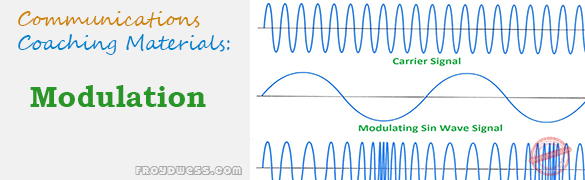This is the Coaching materials in Modulation Part 2 as one topic in ECE Board Exam taken from various sources including but not limited to past Board Examination Questions in Electronic System and Technologies (EST), Communications Books, Journals and other Communications References. This particular Coaching Notes in Communications Engineering has random Questions and Answers in random topics. Make sure to familiarize this review notes to increase the chance of passing the ECE Board Exam.

### Modulation Coaching Materials Part 2

76. Used to suppress carrier in single sideband transmitters.

Ans. balance modulator *

77. Carrier is said to be overmodulated if the positive peak rises to a value _____ of the maximum unmodulated carrier.

Ans. more than twice *

78. Class of bias produce least harmonics

Ans. class A *

79. Devices used to make modulated envelope visible.

Ans. oscilloscope *

80. What will be the result in balanced modulation if not perfectly balanced.

Ans. the carrier is transmitted *

Ans. generate high power *

82. Filter attenuates signals, passes below and above that band.

Ans. band stop *

83. To provide 2 or more voice currents with same carrier.

Ans. ISM emission *

84. To raise the power levels of AM signals, the class of amplifier used is _____.

Ans. class A *

85. Supposed a voice frequency of 400 Hz is transmitted on an AM radio station operating on 590 kHz, the voice frequency 400 Hz is NOT the ______ frequency.

Ans. modulated *

86. What will normal AM receiver detect from an unmodulated RF AC wave?

Ans. nothing *

87. Splatter is the result of ________.

Ans. overmodulation *

88. What happens in standard AM transmission, no modulating signal is being transmitted?

Ans. there are no sidebands *

89. B8E, form of modulation also known as ______.

Ans. Independent Sideband Transmission *

90. Colloquial term describes additional side frequencies produced by overmodulation or distortion in AM.

Ans. splatter *

91. Shape trapezoidal pattern at 100% modulation.

Ans. triangle

92. What is the effect if the gain level being too high for signals entering the modulator?

Ans. distortion and splatter *

93. The RF signal produce; carrier frequency (fc) minus modulating frequency (fm).

Ans. LSB *

94. Mixer is also known as:

Ans. converter *

95. In filter design, the maximum SB suppression is:

Ans. 50 dB *

96. Transmitter power output in SSB operation is expressed in terms of

Ans. PEP *

97. For SSB transmitter, the average power is typically _____ of the peak envelope power, with the typical human speech.

Ans. 1/4to 1/3 *

98. Modulation system most noise resistant

Ans. FM *

99. Pre-emphasis provides extra noise immunity by

Ans. converting phase modulation to FM *

100. The three major types of demodulators:

101. In a frequency synthesizer, smallest amount which output frequency can be changed?

Ans. resolution *

102. Internal capacitance, causes feedback produces same effect on…

Ans. Miller effect *

103. Small length of wire found in some RF equipment, connected only at one end and use as a capacitance to ground.

Ans. gimmick *

104. Movement of signal from one frequency to another using mixer-oscillator combination.

Ans. frequency translation *

105. Feature of modulating tone, FM deviation is proportional

Ans. amplitude *

106. Modulating 2 waves of the same frequency, but with _____ phase difference is equivalent to modulating both amplitude and phase of the same carrier.

Ans. 90 degrees *

107. Frequency of unmodulated carrier of an FM.

Ans. rest frequency *

108. What determines stations that will be selected by a tuner?

Ans. resonant frequency of tuner *

109. Periodic waveforms consist of add harmonics.

Ans. square wave *

110. Major problem with VHF oscillator.

Ans. poor frequency stability *

111. What happens to a spectrum of repetitive pulse as the pulse width decrease?

Ans. more harmonics of the same phase *

112. Keyed AGC is AGC that

Ans. is used in TV receivers *

113. Main disadvantage of single-tube transmitter.

Ans. frequency instability *

114. What is reduced by rounding off square wave emission.

Ans. bandwidth *

115. Gained by operating oscillator on some subharmonic of frequency.

Ans. frequency stability *

116. Multiplexing, oldest and simplest.

Ans. space division multiplexing *

117. Device display signal state of many lines simultaneously.

Ans. logic analyzer *

118. Most common IF carrier frequency.

Ans. 70 MHz *

119. Citizen Band (CB) Radio Service is a two-way voice communication device, it uses frequency range from ______ MHz.

Ans. 26.965 to 27.405 *

120. What determines the rate of frequency swing for an FM broadcast transmitter?

Ans. modulation frequency *

121. In PLL demodulating an FM signal.

Ans. VCOout = FMin *

122. In PLL frequency modulator, fm

Ans. error signal *

123. Find the modulation index if a 10 V carrier is amplitude-modulated by three different frequencies with amplitudes of 1 V, 2 V, and 3 V, respectively.

Ans. 0.374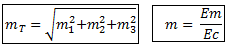124. Calculate the modulation index for a waveform with a maximum voltage of 150 V and minimum voltage of 70 V.

Ans. 0.364 or 36.4%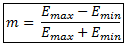125. An AM broadcast transmitter has a carrier power output of 50 kW. What is the total power would be produced with 80% modulation?

Ans. 66 kW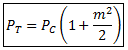126. An AM broadcast transmitter has a carrier power output of 50 kW. What is the power in one sideband with 80% modulation?

Ans. 8 kW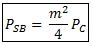127. An AM broadcast transmitter has a carrier power output of 50 kW. What is the total sideband power with 80% modulation?

Ans. 16 kW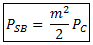128. An AM broadcast transmitter radiates 66 kW power when 100% modulated. If the carrier and one sideband is suppressed, how much power is save?

Ans. 55 kW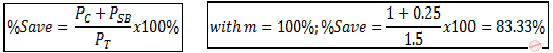129. A transmitter with 10 kW carrier power transmit 12 kW when modulated with a single sine wave. When modulated with another sinewave at 50% modulation, calculate the total transmitted power.

Ans. 13.25 *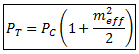130. The rms antenna current of a transmitter is 10 A when unmodulated, it increases by 2 A when modulated. Calculate the modulation index.

Ans. 93.8% *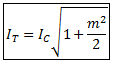131. In SSBSC system, if the peak envelope power (PEP) is 10 W, what will be the value of the maximum instantaneous peak power?

Ans. 20 W *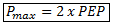132. In SSBSC system, if the peak voltage is 25 volts and the load resistance is 50 ohms, what will be the value of the peak envelope power (PEP)?

Ans. 6.25 W *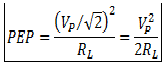133. A carrier wave with an RMS voltage of 20 V and a frequency = 1.5 MHz, is modulated by a sine wave with a frequency of 500 Hz and amplitude of 10 VRMS. Determine the peak voltage of the carrier and of the lower side frequency.

Ans. 28.3 V; 7.1 V *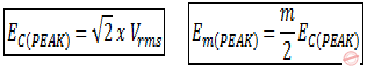134. An FM modulator has a modulator deviation constant of kf = 30 kHz/V and operates at a carrier frequency of 175 MHz. Find the output frequency instantaneous value of the modulating signal equal to -2V.

Ans. 174.94 MHz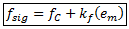135. An FM modulator has a modulator deviation constant of kf = 30 kHz/V and operates at a carrier frequency of 175 MHz is modulated by a 3 V sine wave. Calculate the deviation.

Ans. 127.2 kHz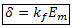136. An FM broadcast transmitter operates at its maximum deviation of 75 kHz. Find the modulation index for a sinusoidal modulation signal with a frequency of 50 Hz.

Ans. 1500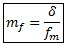137. A phase modulator has a phase modulator sensitivity of kp = 2 radians per volt. What RMS voltage of a sine wave would cause a peak phase deviation of 60 degrees?

Ans. 0.37 V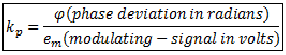138. An FM communications transmitter has a maximum frequency deviation of 5 kHz and a range of modulating frequencies from 300 Hz to 3 kHz. What is the maximum phase shift that it produces?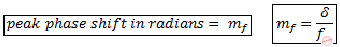139. An FM communications transmitter has a maximum frequency deviation of 5 kHz and a range of modulating frequencies from 300 Hz to 3 kHz. What is the minimum phase shift that it produces?

140. A phase modulation system, has a modulation index mp = 1.5, what is maximum phase shift?

Ans. 86 degrees *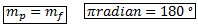141. A phase modulator has a sensitivity of kp = 3 radians per volt. How much frequency deviation does it produces with a sine-wave input of 2 V peak at a frequency of 1 kHz?

Ans. 6 kHz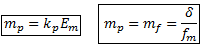142. An FM signal has a deviation of 3 kHz and a modulating frequency of 1 kHz. Its total power is 5 W, developed across a 50-ohm resistive load. The carrier frequency is 160 MHz. Using Carson’s rule, calculate the bandwidth of the signal.

Ans. 16 kHz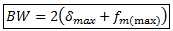143. An FM signal has a modulation index, mf = 3 and a modulating frequency of 2 kHz. Its total power is 5W, developed across a 50-ohm resistive load. The carrier frequency is 160 MHz. Using Carson’s rule, calculate the bandwidth of the signal.

Ans. 16 kHz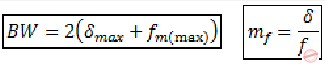144. An FM signal has a frequency deviation of 5 kHz and a modulating frequency of 1 kHz. The signal to noise ratio at the input to the receiver detector is 20 dB. Calculate the approximate signal-to-noise ratio at the detector output.

Ans. 34 dB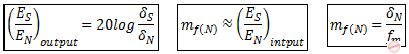145. A crytal oscillator is accurate within 0.0005%. How far off frequency could be at 27 MHz?

Ans. 135 Hz

146. A transmitter has a carrier power output of 10 W at an efficiency of 70%. How much power must be supplied by the modulating amplifier for 100% modulation?

Ans. 7.14 W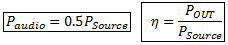147. A transmitter operates from a 12 V supply, with a collector current of 2 A. The modulation transformer has a turns ratio of 4:1. What is the load impedance seen by the audio amplifier?

Ans. 96 ohms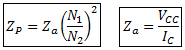148. A collector modulated class C amplifier has a carrier output power Pc of 100 W and an efficiency of 70%. Calculate the supply power and the transistor power dissipation with 100% modulation.

Ans. Ps = 214 W; Pd= 64 W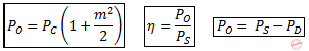149. An AM transmitter is required to produce 10 W of carrier power when operating from a 15 V supply. What is the required load impedance as seen from the collector?

Ans. 11.25 ohms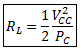150. A portable radio transmitter has to operate transmitter has to operate at temperature from -5 to 35 degrees C. If its signal is derived from a crystal oscillator with a temperature coefficient of +1 ppm per degree Celsius and it transmits at exactly 146 MHz at 20 degrees C, find the transmitting frequency at the upper temperature limit.

Ans. 146.00219 MHz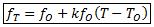151. A phase-locked loop has a VCO with a free-running frequency of 12 MHz. As the frequency of the reference input is gradually raised from zero, the loop locks at 10 MHz and comes out again at 16 MHz. Find the capture range and lock range.

Ans. Capture range = 4 MHz, Lock range = 8 MHz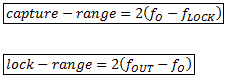### Important List of Communications Engineering Materials

Help Me Makes a Difference!

 P inoyBIX educates thousands of reviewers/students a day in preparation for their board examinations. Also provides professionals with materials for their lectures and practice exams. Help me go forward with the same spirit. “Will you make a small \$5 gift today?” Option 1 : \$1 USD Option 2 : \$3 USD Option 3 : \$5 USD Option 4 : \$10 USD Option 5 : \$25 USD Option 6 : \$50 USD Option 7 : \$100 USD Option 8 : Other Amount#### One Response

1.Rishvan Gunalan
© 2014 PinoyBIX Engineering. © 2019 All Rights Reserved | How to Donate? |#### GEAS Solution

Dynamics problem Economics problem Physics problem Statics problem Strength problem Thermodynamics problem

#### Questions and Answers in GEAS

Engineering Economics Engineering Laws and Ethics Engineering Management Engineering Materials Engineering Mechanics General Chemistry Physics Strength of Materials Thermodynamics
Consider Simple Act of Caring!: LIKE MY FB PAGE

Our app is now available on Google Play, Pinoybix Elex